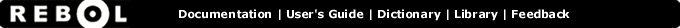# Negate - Function Summary

## Summary:

Changes the sign of a number.

negate number

## Arguments:

number - The number argument. (must be: number pair money time bitset)

## Description:

Returns the negative of the value provided.

 ``` print negate 123 -123```

 ``` print negate -123 123```

 ``` print negate 123.45 -123.45```

 ``` print negate -123.45 123.45```

 ``` print negate 10:30 -10:30```

 ``` print negate 100x20 -100x-20```

 ``` print negate 100x-20 -100x20```

## Related:

+ - Returns the result of adding two values.
- - Returns the second value subtracted from the first.
negative? - Returns TRUE if the number is negative.
positive? - Returns TRUE if the value is positive.